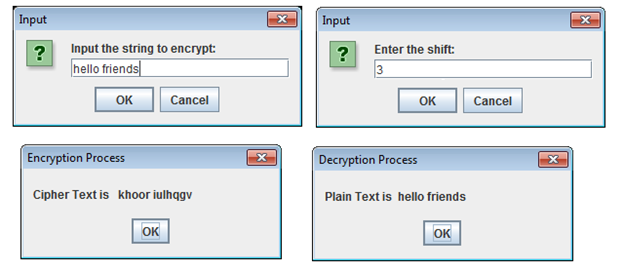Mono-Alphabetic cipher also known relies on a fixed replacement structure. In this technique each alphabet in the plain text can be replaced randomly, i.e. there is no relationship between the alphabets been replaced.

In the program we are implementing Mono-Alphabetic cipher which is an example of substitution cipher. Program consists of two methods encryption and decryption. The encryption method has two parameter one the plain text and second is key. In Mono-Alphabetic cipher each alphabet of plain text is replaced by other alphabet. For e.g. in a given plain text message each A can be replaced with any other alphabet i.e. B through Z, each alphabet B can be replaced by any letter i.e. A or C to Z & so on. The decryption method also has two parameters one encrypted message and key. It does opposite process of encryption.

Program

importjavax.swing.JOptionPane;

public class MonoAlphabetic

{

public static void main(String[] args){

// TODO code application logic here

String plain_text,cipher1,cipher2;

plain_text=JOptionPane.showInputDialog(“Input the string to encrypt:”);

int shift=Integer.parseInt(JOptionPane.showInputDialog(“Enter the shift:”));

cipher1=encryption(plain_text,shift);

JOptionPane.showMessageDialog (null, “Cipher Text is   ” + cipher1, “Encryption Process”, JOptionPane.PLAIN_MESSAGE);

cipher2=decryption(cipher1,shift);

JOptionPane.showMessageDialog (null, “Plain Text is  ” + cipher2, “Decryption Process”, JOptionPane.PLAIN_MESSAGE);

}

public static String encryption(String cipher,int shift)

{

String result=””;

int offset;

for(int i=0;i<cipher.length();i++)

{

if(cipher.charAt(i)>=’a’&&cipher.charAt(i)<=’z’)

{

offset=((int)(cipher.charAt(i)))-97;

offset=(offset+shift)%26;

result+=(char)(offset+97);

}

else if(cipher.charAt(i)>=’A’&&cipher.charAt(i)<=’Z’)

{

offset=((int)(cipher.charAt(i)))-65;

offset=(offset+shift)%26;

result+=(char)(offset+65);

}

else

result=result+cipher.charAt(i);

}

return result;

}

public static String decryption(String plain,int shift)

{

String result=””;

int offset;

for(int i=0;i<plain.length();i++)

{

if(plain.charAt(i)>=’a’&&plain.charAt(i)<=’z’)

{

offset=((int)(plain.charAt(i)))-97;

offset=(offset-shift)%26;

if(offset<0)

offset+=26;

result+=(char)(offset+97);

}

else if(plain.charAt(i)>=’A’&&plain.charAt(i)<=’Z’)

{

offset=((int)(plain.charAt(i)))-65;

offset=(offset-shift)%26;

if(offset<0)

offset+=26;

result+=(char)(offset+65);

}

else

result=result+plain.charAt(i);

}

return result;

}}